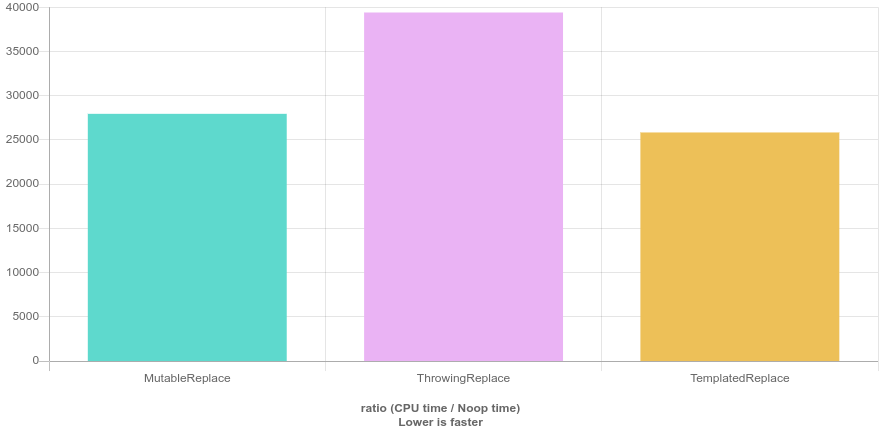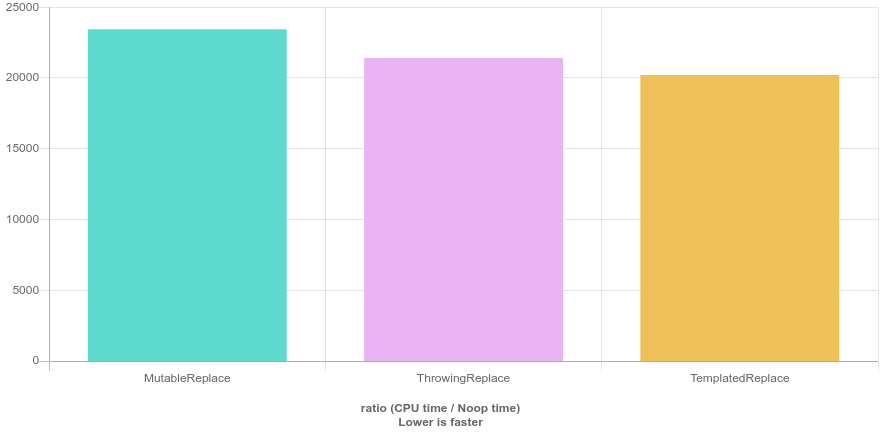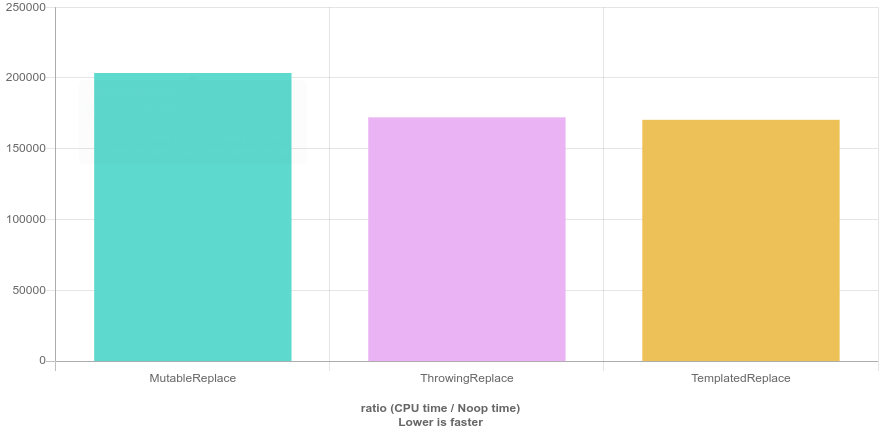Recently in my series on C++ algorithms, I presented the different replace functions and said that they will replace all the matching elements. If you want to replace only one element or n elements, you have to find another way.

But what’s that other way?

## Mutable lambdas scanning all the way through

One of the readers, Ali, left his solution in the comments section. Thank you, Ali!

std::vector<int> numbers { 1, 2, 3, 4, 5, 4, 7, 4, 9, 10 };
std::replace_if(numbers.begin(), numbers.end(), [i = 0](auto number) mutable {return number == 4 && i++ < 2;}, 42);


This is definitely something we can do, and if rename variable i to something like alreadyReplaced, it becomes even more readable.

Of course, we can slightly change the solution to use a named lambda or even a function object keeping it essentially the same.

They still share the same common disadvantage. They will iterate through the whole input container. This might or might not be an issue depending on your use case, the size of the container, etc. But if you have a container of thousands of elements or more, it’ll likely be a problem.

In addition, using mutable in lambdas are not very elegant. In a functional programming style - and that’s pretty much what the STL is about -, a function should always produce the same output given the same input. If we have mutable lambdas, most probably it’ll not be the case (or the mutable would be completely superfluous).

## Still mutable, but throwing

If we accept to have a mutable lambda and while we avoid scanning all the elements after having replaced enough of them, we could also throw an exception. If you came to C++ after having coded in Python, this might seem completely valid for you, but in C++ it’s not the best idea to use exceptions in a nominal control flow. And let’s be fair, throwing an exception if you replaced n elements when you wanted to replace exactly n elements, it’s not an exceptional event.

But let’s see how it would be used.

try {
std::replace_if(numbers.begin(), numbers.end(), [i = 0](auto number) mutable {
if (i == 2) {
throw std::invalid_argument{"Already replaced " + std::to_string(i) + " elements"};
}
return number == 4 && i++ < 2;
}, 42);
} catch (const std::exception& ex) {
std::cout << "Done with replacing: " << ex.what() << std::endl;
}


At the end of the article, we’ll see what Quick Bench says about the performance of the different versions.

Whatever we are going to see performance-wise, there might be other restrictions in your project. You might discourage/ban the usage of exceptions in your code like Google used to do. You also have to consider those.

Now, let’s look for another solution.

## Use other STL algorithms

If we wanted to use only algorithms we could do something similar:

  std::vector<int> numbers { 1, 2, 3, 4, 5, 4, 7, 4, 9, 10 };
auto pos = std::find(numbers.begin(), numbers.end(), 4);
std::replace(pos, pos+1, 4, 42);


First, we find the first occurrence of 4, which is the element we look for and then we call the replace algorithm on that exact position.

The good parts are that we use only STL algorithms, so we stay on the same level of abstraction and in the same style. On the other hand, we have that small, but still existing overhead that comes with calling an algorithm, plus we make an extra comparison whereas we could write only this:

  std::vector<int> numbers { 1, 2, 3, 4, 5, 4, 7, 4, 9, 10 };
auto pos = std::find(numbers.begin(), numbers.end(), 4);
*pos=42;


If we want to replace the n first elements, we have to repeat the same block n times.

In C++, there is nothing like n.times in Ruby, so we have to use a for loop here.

  std::vector<int> numbers { 1, 2, 3, 4, 5, 4, 7, 4, 9, 10 };
size_t n = 2;
for (size_t i = 0; i < n; ++i) {
auto pos = std::find(numbers.begin(), numbers.end(), 4);
*pos=42;
}


Each time we look for an element that matches our predicate, then we replace it by 42.

This is not efficient because we always look from the beginning of the input container, whereas we know that there should be no elements matching before what we already replaced. (For simplicity, we ignore the case of concurrent updates in this article).

To overcome this deficiency, we can create a variable begin that will mark the beginning point of our search. Before we start the loop, it points to the beginning of the container and then at each iteration it is updated with the result of std::find. And in fact, it would be correct to advance the begin variable by one before starting over with the next iteration as we don’t need to compare against what we just updated.

#include <algorithm>
#include <iostream>
#include <vector>

int main() {
std::vector<int> numbers { 1, 2, 3, 4, 5, 4, 7, 4, 9, 10 };
size_t n = 2;
auto begin = numbers.begin();
for (size_t i = 0; i < n; ++i) {
begin = std::find(begin, numbers.end(), 4);
std::replace(begin, begin+1, 4, 42);
}

std::cout << " copied numbers: ";
for (const auto& number : numbers) {
std::cout << ' ' << number;
}
std::cout << '\n';

return 0;
}


At this point, it seems we have something useable, and readable. Let’s move it to its own function.

std::vector<int>::iterator replace_n(std::vector<int>::iterator begin, std::vector<int>::iterator end, int oldValue, int newValue, size_t n) {
for (size_t i = 0; i < n; ++i) {
begin = std::find(begin, end, 4);
std::replace(begin, begin+1, 4, 42);
}
return begin;
}

// ...
std::vector<int> numbers { 1, 2, 3, 4, 4, 5, 4, 7, 4, 9, 10 };
replace_n(numbers.begin(), numbers.end(), 4, 42, 2);



Now it’s quite neat, both the naming and the interface matches what we are used in the STL.

The only problem is that this function is not at all reusable. Both the container and the contained types are fixed. Let’s change this!

template <typename T, typename Iter>
Iter replace_n(Iter begin, Iter end, T oldValue, T newValue, size_t n) {
for (size_t i = 0; i < n; ++i) {
begin = std::find(begin, end, 4);
std::replace(begin, begin+1, 4, 42);
}
return begin;
}


Now we have something that we can use on any iterable container with any type that defines an operator==. The only problem here is that T and Iter doesn’t have to correspond to each other. In practice, it means that you can pass in a vector of integers while you want to change a string value with another string.

With type traits or concepts this problem is solvable, but it goes beyond the scope of this article. We stop at this point, with this implementation.

## Performance

Where do we stand performance-wise?

The pictures are always showing the unoptimized values.

With a small number of elements (100) the fastest is our final solution. It’s about 10% better than the original one using mutable lambdas and 40% better than the throwing one. Using optimization the difference between mutable and templated vanishes.On a thousand elements, the effect of scans kicks in and makes throwing a bit faster than the mutable version. But that difference goes away with optimization. The final templated solution beats the others by 10-20 percent.When moving up to 10000 elements, the difference between the mutable and the throwing version stabilizes, with the templating still a little bit faster.What we can see is that these differences are not significant. You won’t solve bottleneck issues, but in all cases, our final solution was at least a little bit faster than the others.

## Conclusion

The problem we solved today is how to replace not all but just n elements of a container. We started with a quite concise solution where we still used std::replace with a mutable lambda that can count how many elements were already replaced. Sadly, it continues the iteration even after having replaced enough elements.

This problem we could solve by throwing an exception, even though in C++ this is clearly not the best way to go. Exceptions are for exceptional events not for general control flow.

Using std::find within a for loop solved all our issues. No extra scans, no exceptions. The price is a raw loop. Thanks to the lack of extra scans and exceptions, it’s also faster than the others, even though the differences are not significant.

Given all that we saw, I would go with the final solution if I needed a replace_n functionality.

Happy coding!

P.S. We can achieve the same output with the ranges library, but that’s a story for another day